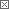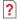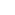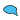Previous in Forum: Circuit Design & execute Next in Forum: ACS800 tripped due to stallfaultMember

Join Date: Jan 2009
Posts: 7

#electrical calculation of total current of 380v and 220v 3phase supply

01/10/2009 1:55 PM

Sir, i want to calculate the total load in current (ampere) for the following voltages supply. In our place we have two different types of voltage supply namely: 1) is the 3 phase 380v which has a line to neutral or ground voltage of 220v and a phase to phase voltages of 380v. and 2) is the 3 phase 220v which has a line to neutral of 110v and phase to phase of 220v. They told me that the formula of getting the total current in amp is I= total watts x power factor divided by square root of 3 x 220v.. my question is where did 220v came from.. and how do you apply this formula to what i said 3 phase 380v and 3 phase 220v power supply 60hz. please advise me with the correct formula or are there any formula in computing total current to get the main amp. (i.e main breaker supply of a load)

Interested in this topic? By joining CR4 you can "subscribe" to
Guru

Join Date: Jun 2008
Location: Alabama
Posts: 1610#1

###Re: electrical calculation of total current of 380v and 220v 3phase supply

01/10/2009 4:08 PM

The formula is amps = wattage/(1.73 x voltage). If the 380 volt and 220 volt are separate services, then determine the wattage for each voltage and substitute it into the formula. Total amps would be the addition of the two. If the 380 volt service feeds the 220 volt service via a transformer then just use the 380 volts in the formula to get the total amps.

Anonymous Poster#2

###Re: electrical calculation of total current of 380v and 220v 3phase supply

01/11/2009 9:36 AM

to calculate the current in amp there is only 2 cases :

1- in 3 phases system, voltage = 380V

the power in Watt is P= (square root of 3)x380x(current in amp)x(power factor)

2- in Single phase, voltage = 220V

the power in Watt is P= 220x(current in amp)

so in the 2 conditions you can find the current in amp

Off Topic (Score 5)
Member

Join Date: Jan 2009
Location: Palestine
Posts: 6#3

###Re: electrical calculation of total current of 380v and 220v 3phase supply

01/11/2009 5:27 PM

The correct formula is:

for 380/3Ph/60Hz and 220/3/60

P(3PH)=sqrt(3)*I*V*Pf*Eff

where P: Power, I: Current, V: Voltage, Pf: power factor, Eff: Effeciency

so I=P/(sqrt(3)*V*Pf*Eff)

for 220V/1Ph/60Hz which came from 380V line and Neutral

and 110 or 120V/1Ph/60Hz which came from 220V line and Neutral

P=I*V*Pf*Eff

so I=P/(V*Pf*Eff)

Note I=I1+I2+....In sum of loads current in each circuit alone

or Itotal=Ptotal/.....as above

to select the Main Circuit Breaker use this formula below:

MOCP=2.25*Imax+the other load Amps, Maximum Operating Current Protection

you can choose MCB which is the next lower value of MOCP

Anonymous Poster#4

###Re: electrical calculation of total current of 380v and 220v 3phase supply

01/12/2009 3:12 PM

al6776bert; your 220 volt 3 phase with a neutral would have a 127 volt from neutral to any phase. perry